Next: About this document ... Up: Waves in Warm Plasmas Previous: Parallel Wave Propagation

Perpendicular Wave Propagation

Let us now consider wave propagation, through a warm plasma, perpendicular to the equilibrium magnetic field. For perpendicular propagation,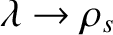, and, hence, from Eq. (1066),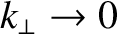. Making use of the asymptotic expansions (1067)-(1068), the matrix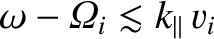simplifies considerably. The dispersion relation can again be written in the form (1074), where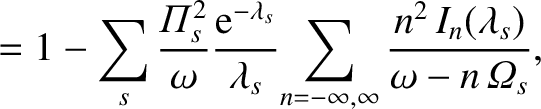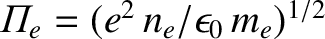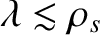(1083)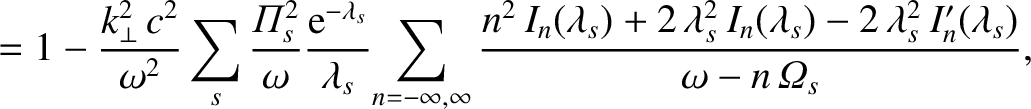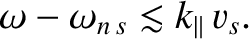(1084)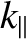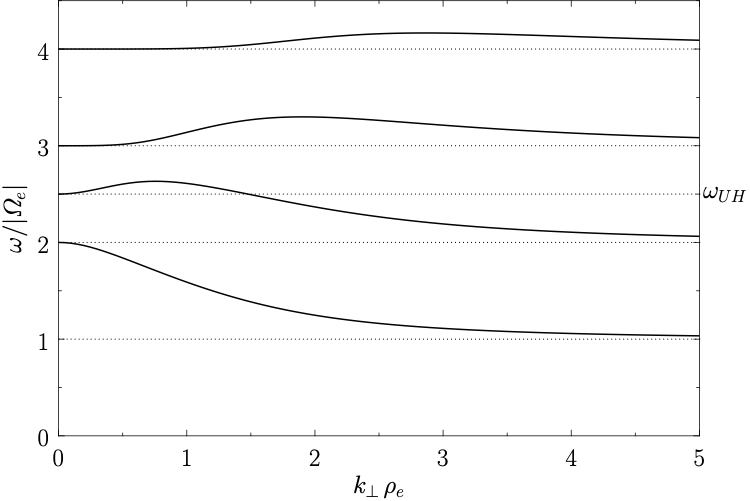(1085)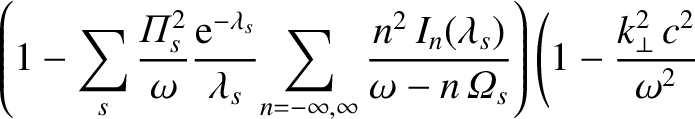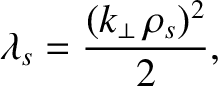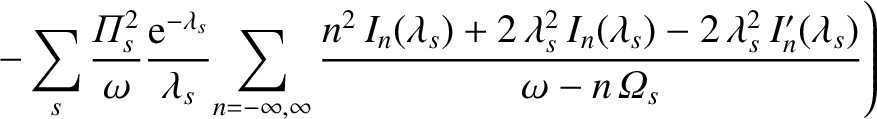(1086)

and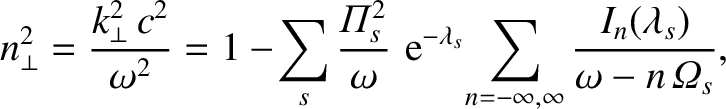. Here,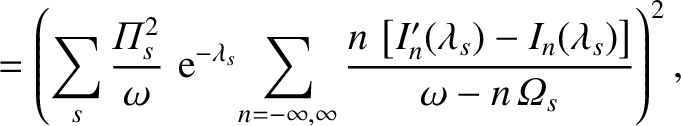(1087)

where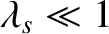is the species-Larmor radius.

The first root of the dispersion relation (1074) is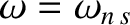(1088)

with the eigenvector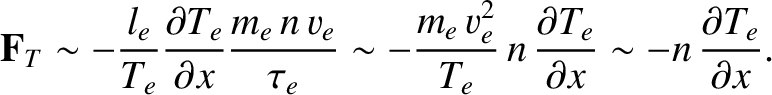. This dispersion relation obviously corresponds to the electromagnetic plasma wave, or ordinary mode, discussed in Sect. 4.10. Note, however, that in a warm plasma the dispersion relation for the ordinary mode is strongly modified by the introduction of resonances (where the refractive index,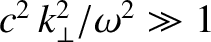, becomes infinite) at all the harmonics of the cyclotron frequencies: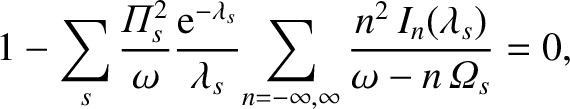(1089)

where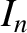is a non-zero integer. These resonances are a finite Larmor radius effect. In fact, they originate from the variation of the wave phase across a Larmor orbit. Thus, in the cold plasma limit,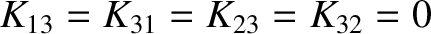, in which the Larmor radii shrink to zero, all of the resonances disappear from the dispersion relation. In the limit in which the wave-length,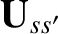, of the wave is much larger than a typical Larmor radius,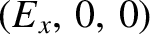, the relative amplitude of theth harmonic cyclotron resonance, as it appears in the dispersion relation (1088), is approximately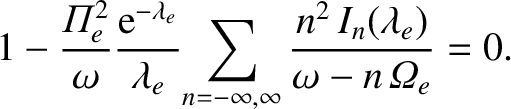[see Eqs. (1069) and (1087)]. It is clear, therefore, that in this limit only low-order resonances [i.e.,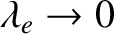] couple strongly into the dispersion relation, and high-order resonances (i.e.,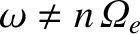) can effectively be neglected. As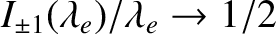, the high-order resonances become increasigly important, until, when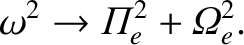, all of the resonances are of approximately equal strength. Since the ion Larmor radius is generally much larger than the electron Larmor radius, it follows that the ion cyclotron harmonic resonances are generally more important than the electron cyclotron harmonic resonances.

Note that the cyclotron harmonic resonances appearing in the dispersion relation (1088) are of zero width in frequency space: i.e., they are just like the resonances which appear in the cold-plasma limit. Actually, this is just an artifact of the fact that the waves we are studying propagate exactly perpendicular to the equilibrium magnetic field. It is clear from an examination of Eqs. (1064) and (1066) that the cyclotron harmonic resonances originate from the zeros of the plasma dispersion functions. Adopting the usual rule that substantial damping takes place whenever the arguments of the dispersion functions are less than or of order unity, it is clear that the cyclotron harmonic resonances lead to significant damping whenever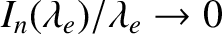(1090)

Thus, the cyclotron harmonic resonances possess a finite width in frequency space provided that the parallel wave-number,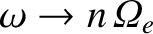, is non-zero: i.e., provided that the wave does not propagate exactly perpendicular to the magnetic field.

The appearance of the cyclotron harmonic resonances in a warm plasma is of great practical importance in plasma physics, since it greatly increases the number of resonant frequencies at which waves can transfer energy to the plasma. In magnetic fusion these resonances are routinely exploited to heat plasmas via externally launched electromagnetic waves. Hence, in the fusion literature you will often come across references to third harmonic ion cyclotron heating'' or second harmonic electron cyclotron heating.''

The other roots of the dispersion relation (1074) satisfy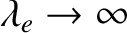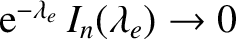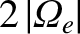(1091)

with the eigenvector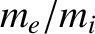. In the cold plasma limit,, this dispersion relation reduces to that of the extraordinary mode discussed in Sect. 4.10. This mode, for which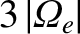, unless the plasma possesses a thermal velocity approaching the velocity of light, is little affected by thermal effects, except close to the cyclotron harmonic resonances,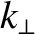, where small thermal corrections are important because of the smallness of the denominators in the above dispersion relation.

However, another mode also exists. In fact, if we look for a mode with a phase velocity much less than the velocity of light (i.e.,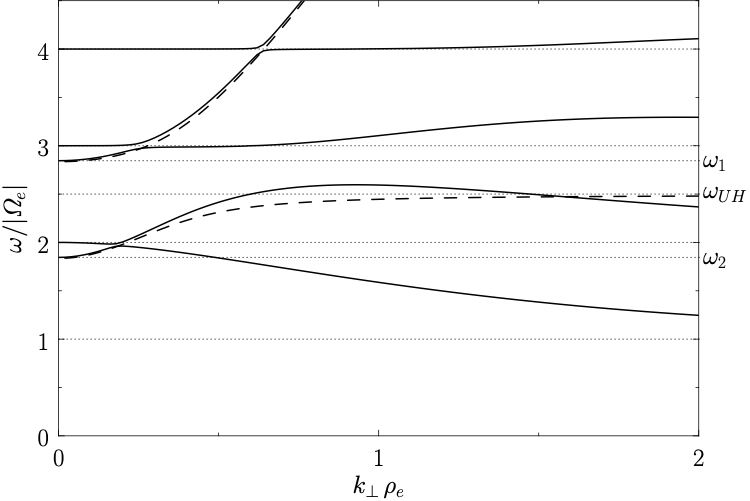) then it is clear from (1083)-(1086) that the dispersion relation is approximately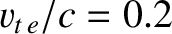(1092)

and the associated eigenvector is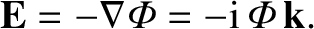. The new waves, which are called Bernstein waves (after I.B. Bernstein, who first discovered them), are clearly slowly propagating, longitudinal, electrostatic waves.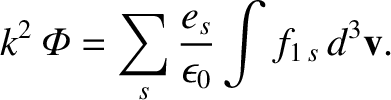Let us consider electron Bernstein waves, for the sake of definiteness. Neglecting the contribution of the ions, which is reasonable provided that the wave frequencies are sufficiently high, the dispersion relation (1092) reduces to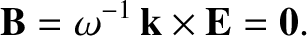(1093)

where the subscriptis dropped, since it is understood that all quantities relate to electrons. In the limit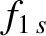(with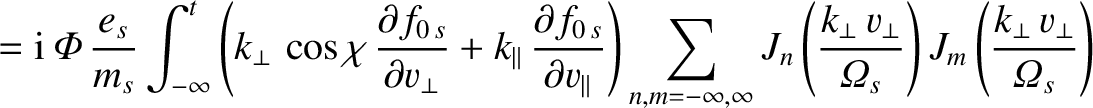), only the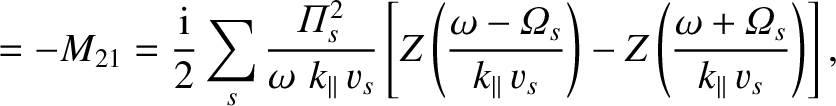terms survive in the above expression. In fact, since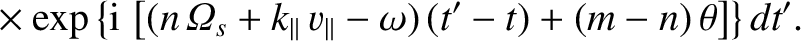as, the dispersion relation yields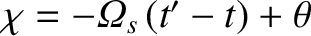(1094)

It follows that there is a Bernstein wave whose frequency asymptotes to the upper hybrid frequency [see Sect. 4.10] in the limit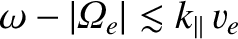. For other non-zero values of, we have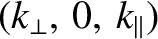as. However, a solution to Eq. (1092) can be obtained if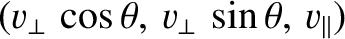at the same time. Similarly, as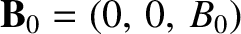we have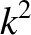. In this case, a solution can only be obtained if, for some, at the same time. The complete solution to Eq. (1092) is sketched in Fig. 38, for the case where the upper hybrid frequency lies between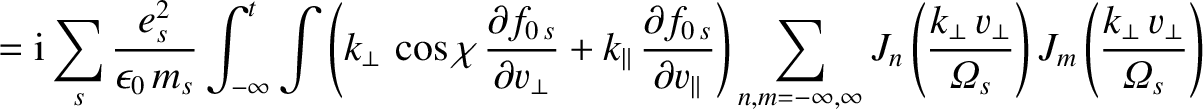and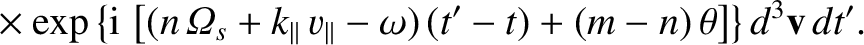. In fact, wherever the upper hybrid frequency lies, the Bernstein modes above and below it behave like those in the diagram.

At small values of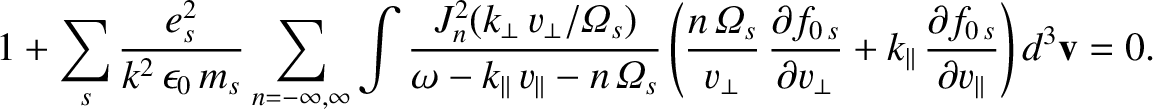, the phase velocity becomes large, and it is no longer legitimate to neglect the extraordinary mode. A more detailed examination of the complete dispersion relation shows that the extraordinary mode and the Bernstein mode cross over near the harmonics of the cyclotron frequency to give the pattern shown in Fig. 39. Here, the dashed line shows the cold plasma extraordinary mode.

In a lower frequency range, a similar phenomena occurs at the harmonics of the ion cyclotron frequency, producing ion Bernstein waves, with somewhat similar properties to electron Bernstein waves. Note, however, that whilst the ion contribution to the dispersion relation can be neglected for high-frequency waves, the electron contribution cannot be neglected for low frequencies, so there is not a complete symmetry between the two types of Bernstein waves.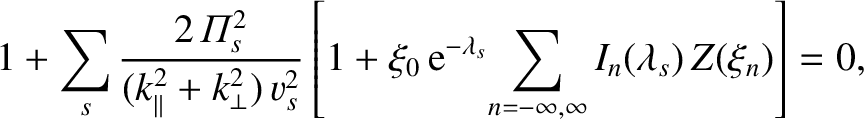Next: About this document ... Up: Waves in Warm Plasmas Previous: Parallel Wave Propagation
Richard Fitzpatrick 2011-03-31